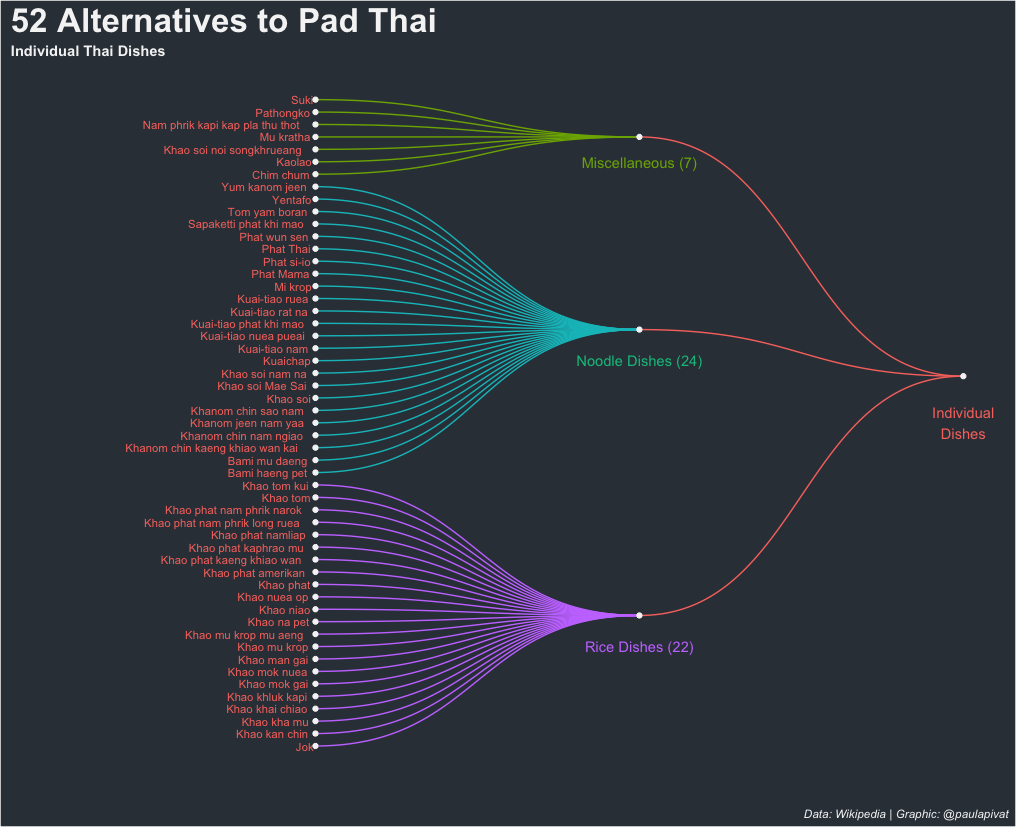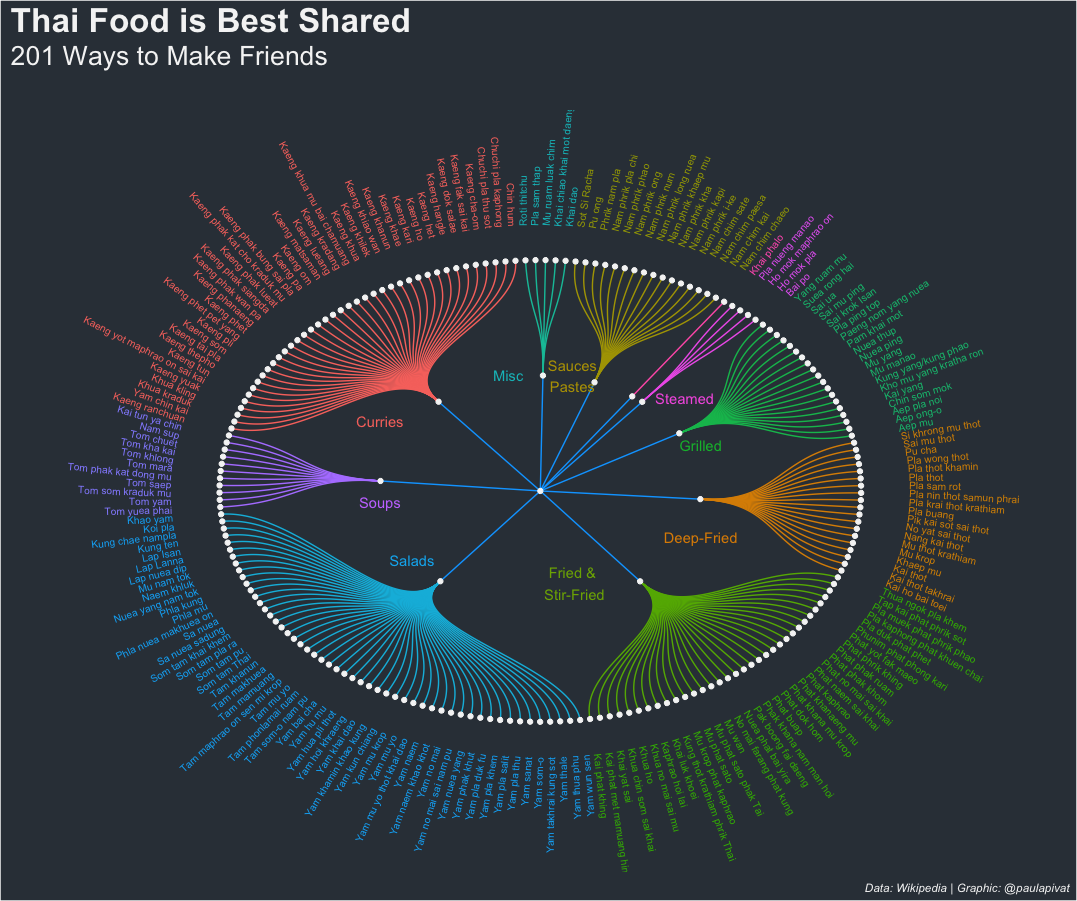# Paul Apivat Data Journey# Visualizing Thai Food with R

This post is Part 2 in the Thai Food Dishes series. Part 1 here.

### Data Cleaning

Data cleaning is typically non-linear.

We'll manipulate the data to explore, learn about the data and see that certain things need cleaning or, in some cases, going back to Python to re-scrape. The columns `a1` and `a6` were scraped differently from other columns due to missing data found during exploration and cleaning.

For certain links, using `.find(text=True)` did not work as intended, so a slight adjustment was made.

For this post, `R` is the tool of choice for cleaning the data.

Here are other data cleaning tasks:

• Changing column names (snake case)
``````# read data

# change column name
df <- df %>%
rename(
Thai_name = `Thai name`,
Thai_name_2 = `Thai name 2`,
Thai_script = `Thai script`,
English_name = `English name`
)
``````
• Remove newline escape sequence (\n)
``````# remove  \n from all columns ----
df\$Thai_name <- gsub("[\n]", "", df\$Thai_name)
df\$Thai_name_2 <- gsub("[\n]", "", df\$Thai_name_2)
df\$Thai_script <- gsub("[\n]", "", df\$Thai_script)
df\$English_name <- gsub("[\n]", "", df\$English_name)
df\$Image <- gsub("[\n]", "", df\$Image)
df\$Region <- gsub("[\n]", "", df\$Region)
df\$Description <- gsub("[\n]", "", df\$Description)
df\$Description2 <- gsub("[\n]", "", df\$Description2)
``````
• Add/Mutate new columns (major_groupings, minor_groupings):
``````# Add Major AND Minor Groupings ----
df <- df %>%
mutate(
major_grouping = as.character(NA),
minor_grouping = as.character(NA)
)
``````
• Edit rows for missing data in Thai_name column: 26, 110, 157, 234-238, 240, 241, 246

Note: This was only necessary the first time round, after the changes are made to how I scraped `a1` and `a6`, this step is no longer necessary:

``````# If necessary; may not need to do this after scraping a1 and a6 - see above
# Edit Rows for missing Thai_name
df[26,]\$Thai_name <- "Khanom chin nam ngiao"
df[110,]\$Thai_name <- "Lap Lanna"
df[157,]\$Thai_name <- "Kai phat khing"
df[234,]\$Thai_name <- "Nam chim chaeo"
df[235,]\$Thai_name <- "Nam chim kai"
df[236,]\$Thai_name <- "Nam chim paesa"
df[237,]\$Thai_name <- "Nam chim sate"
df[238,]\$Thai_name <- "Nam phrik i-ke"
df[240,]\$Thai_name <- "Nam phrik kha"
df[241,]\$Thai_name <- "Nam phrik khaep mu"
df[246,]\$Thai_name <- "Nam phrik pla chi"
``````
• save to "edit_thai_dishes.csv"
``````# Write new csv to save edits made to data frame
write_csv(df, "edit_thai_dishes.csv")
``````

### Data Visualization

There are several ways to visualize the data. Because we want to communicate the diversity of Thai dishes, aside from Pad Thai, we want a visualization that captures the many, many options.

I opted for a dendrogram. This graph assumes hierarchy within the data, which fits our project because we can organize the dishes in grouping and sub-grouping.

#### How might we organized Thai dishes?

We first make a distinction between individual and shared dishes to show that Pad Thai is not even close to being the best individual dish. And, in fact, more dishes fall under the shared grouping.

To avoid cramming too much data into one visual, we'll create two separate visualizations for individual vs. shared dishes.

Here is the first dendrogram representing 52 individual dish alternatives to Pad Thai.Creating a dendrogram requires using the `ggraph` and `igraph` libraries. First, we'll load the libraries and sub-set our data frame by filtering for Individual Dishes:

``````df <- read_csv("edit_thai_dishes.csv")

library(ggraph)
library(igraph)

df %>%
select(major_grouping, minor_grouping, Thai_name, Thai_script) %>%
filter(major_grouping == 'Individual dishes') %>%
group_by(minor_grouping) %>%
count()
``````

We create edges and nodes (i.e., from and to) to create the sub-groupings within Individual Dishes (i.e., Rice, Noodles and Misc):

``````# Individual Dishes ----

# data: edge list
d1 <- data.frame(from="Individual dishes", to=c("Misc Indiv", "Noodle dishes", "Rice dishes"))

d2 <- df %>%
select(minor_grouping, Thai_name) %>%
slice(1:53) %>%
rename(
from = minor_grouping,
to = Thai_name
)

edges <- rbind(d1, d2)

# plot dendrogram (idividual dishes)
indiv_dishes_graph <- graph_from_data_frame(edges)

ggraph(indiv_dishes_graph, layout = "dendrogram", circular = FALSE) +
geom_edge_diagonal(aes(edge_colour = edges\$from), label_dodge = NULL) +
geom_node_text(aes(label = name, filter = leaf, color = 'red'), hjust = 1.1, size = 3) +
geom_node_point(color = "whitesmoke") +
theme(
plot.background = element_rect(fill = '#343d46'),
panel.background = element_rect(fill = '#343d46'),
legend.position = 'none',
plot.title = element_text(colour = 'whitesmoke', face = 'bold', size = 25),
plot.subtitle = element_text(colour = 'whitesmoke', face = 'bold'),
plot.caption = element_text(color = 'whitesmoke', face = 'italic')
) +
labs(
title = '52 Alternatives to Pad Thai',
subtitle = 'Individual Thai Dishes',
caption = 'Data: Wikipedia | Graphic: @paulapivat'
) +
expand_limits(x = c(-1.5, 1.5), y = c(-0.8, 0.8)) +
coord_flip() +
annotate("text", x = 47, y = 1, label = "Miscellaneous (7)", color = "#7CAE00")+
annotate("text", x = 31, y = 1, label = "Noodle Dishes (24)", color = "#00C08B") +
annotate("text", x = 8, y = 1, label = "Rice Dishes (22)", color = "#C77CFF") +
annotate("text", x = 26, y = 2, label = "Individual\nDishes", color = "#F8766D")
``````

#### What is the best way to organized the different dishes?

There are approximately 4X as many shared dishes as individual dishes, so the dendrogram should be circular to fit the names of all dishes in one graphic.

A wonderful resource I use regularly for these types of visuals is the R Graph Gallery. There was a slight issue in how the text angles were calculated so I submitted a PR to fix.

Perhaps distinguishing between individual and shared dishes is too crude, within the dendrogram for 201 shared Thai dishes, we can see further sub-groupings including Curries, Sauces/Pastes, Steamed, Grilled, Deep-Fried, Fried & Stir-Fried, Salads, Soups and other Misc:``````# Shared Dishes ----
df %>%
select(major_grouping, minor_grouping, Thai_name, Thai_script) %>%
filter(major_grouping == 'Shared dishes') %>%
group_by(minor_grouping) %>%
count() %>%
arrange(desc(n))

d3 <- data.frame(from="Shared dishes", to=c("Curries", "Soups", "Salads",
"Fried and stir-fried dishes", "Deep-fried dishes", "Grilled dishes",
"Steamed or blanched dishes", "Stewed dishes", "Dipping sauces and pastes", "Misc Shared"))

d4 <- df %>%
select(minor_grouping, Thai_name) %>%
slice(54:254) %>%
rename(
from = minor_grouping,
to = Thai_name
)

edges2 <- rbind(d3, d4)

# create a vertices data.frame. One line per object of hierarchy
vertices = data.frame(
name = unique(c(as.character(edges2\$from), as.character(edges2\$to)))
)

# add column with group of each name. Useful to later color points
vertices\$group = edges2\$from[ match(vertices\$name, edges2\$to)]

# Add information concerning the label we are going to add: angle, horizontal adjustment and potential flip
# calculate the ANGLE of the labels
vertices\$id=NA
myleaves=which(is.na(match(vertices\$name, edges2\$from)))
nleaves=length(myleaves)
vertices\$id[myleaves] = seq(1:nleaves)
vertices\$angle = 360 / nleaves * vertices\$id + 90

# calculate the alignment of labels: right or left
vertices\$hjust<-ifelse( vertices\$angle < 275, 1, 0)

# flip angle BY to make them readable
vertices\$angle<-ifelse(vertices\$angle < 275, vertices\$angle+180, vertices\$angle)

# plot dendrogram (shared dishes)
shared_dishes_graph <- graph_from_data_frame(edges2)

ggraph(shared_dishes_graph, layout = "dendrogram", circular = TRUE) +
geom_edge_diagonal(aes(edge_colour = edges2\$from), label_dodge = NULL) +
geom_node_text(aes(x = x*1.15, y=y*1.15, filter = leaf, label=name, angle = vertices\$angle, hjust= vertices\$hjust, colour= vertices\$group), size=2.7, alpha=1) +
geom_node_point(color = "whitesmoke") +
theme(
plot.background = element_rect(fill = '#343d46'),
panel.background = element_rect(fill = '#343d46'),
legend.position = 'none',
plot.title = element_text(colour = 'whitesmoke', face = 'bold', size = 25),
plot.subtitle = element_text(colour = 'whitesmoke', margin = margin(0,0,30,0), size = 20),
plot.caption = element_text(color = 'whitesmoke', face = 'italic')
) +
labs(
title = 'Thai Food is Best Shared',
subtitle = '201 Ways to Make Friends',
caption = 'Data: Wikipedia | Graphic: @paulapivat'
) +
#expand_limits(x = c(-1.5, 1.5), y = c(-0.8, 0.8)) +
expand_limits(x = c(-1.5, 1.5), y = c(-1.5, 1.5)) +
coord_flip() +
annotate("text", x = 0.4, y = 0.45, label = "Steamed", color = "#F564E3") +
annotate("text", x = 0.2, y = 0.5, label = "Grilled", color = "#00BA38") +
annotate("text", x = -0.2, y = 0.5, label = "Deep-Fried", color = "#DE8C00") +
annotate("text", x = -0.4, y = 0.1, label = "Fried &\n Stir-Fried", color = "#7CAE00") +
annotate("text", x = -0.3, y = -0.4, label = "Salads", color = "#00B4F0") +
annotate("text", x = -0.05, y = -0.5, label = "Soups", color = "#C77CFF") +
annotate("text", x = 0.3, y = -0.5, label = "Curries", color = "#F8766D") +
annotate("text", x = 0.5, y = -0.1, label = "Misc", color = "#00BFC4") +
annotate("text", x = 0.5, y = 0.1, label = "Sauces\nPastes", color = "#B79F00")
``````

For more content on data science, R, Python, SQL and more, find me on Twitter.